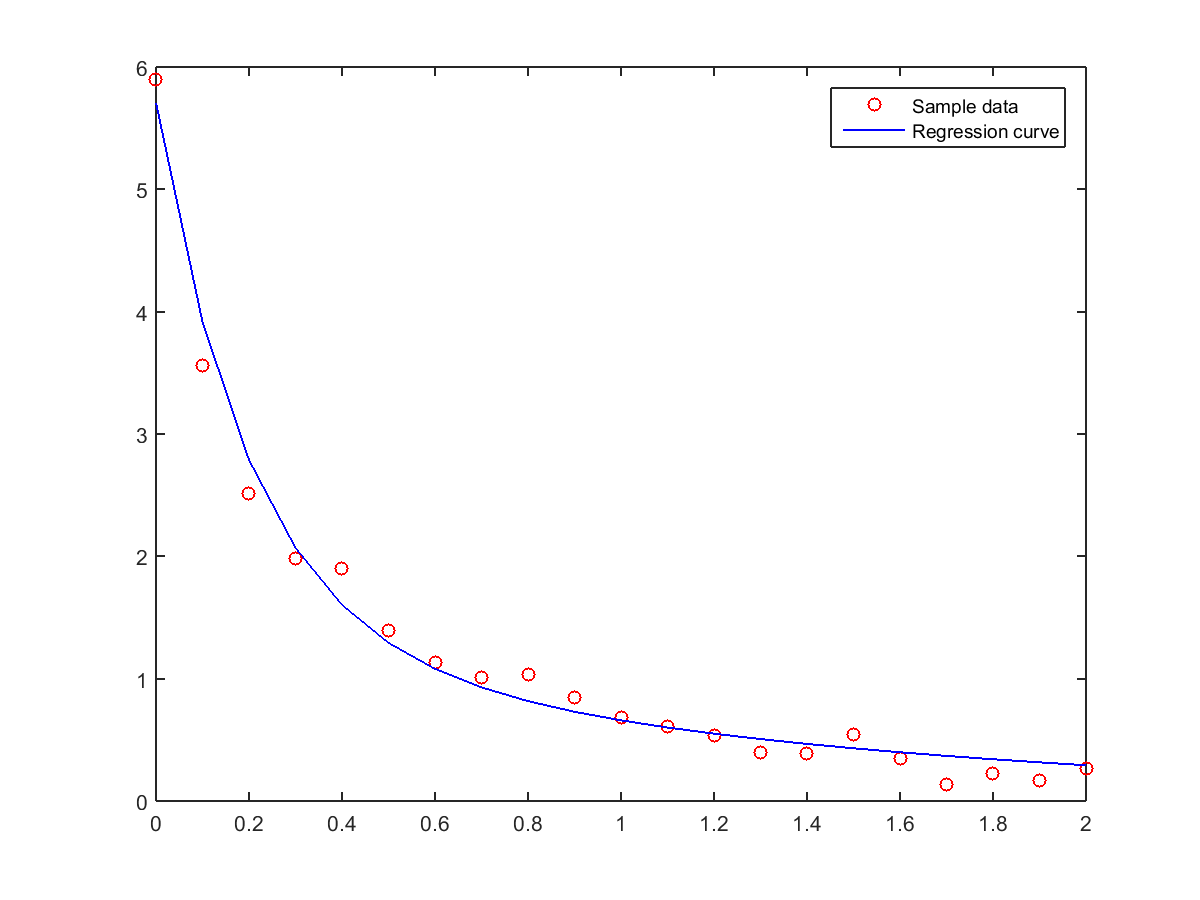## 10-3 ?䂿??扯艘甇賂?雿輻鍂 fminsearch

• 無法一次找到最佳解。
• 無法保證能夠找到最佳解。
• 須引用各種非線性最佳化的方法。
• 各種相關數學性質並不明顯。

$$y= a_1 e^{\lambda_1 x} + a_2 e^{\lambda_2 x}$$

Example 1: 10-曲線擬合與迴歸分析/errorMeasure1.mfunction squaredError = errorMeasure1(theta, data) if nargin<1; return; end x = data(:,1); y = data(:,2); y2 = theta(1)*exp(theta(3)*x)+theta(2)*exp(theta(4)*x); squaredError = sum((y-y2).^2);

Example 2: 10-曲線擬合與迴歸分析/nonlinearFit01.mload data.txt theta0 = [0 0 0 0]; tic theta = fminsearch(@(x)errorMeasure1(x, data), theta0); fprintf('計算時間 = %g\n', toc); x = data(:, 1); y = data(:, 2); y2 = theta(1)*exp(theta(3)*x)+theta(2)*exp(theta(4)*x); plot(x, y, 'ro', x, y2, 'b-'); legend('Sample data', 'Regression curve'); fprintf('誤差平方和 = %d\n', sum((y-y2).^2));計算時間 = 0.0762827 誤差平方和 = 5.337871e-01MATLAB程式設計：進階篇